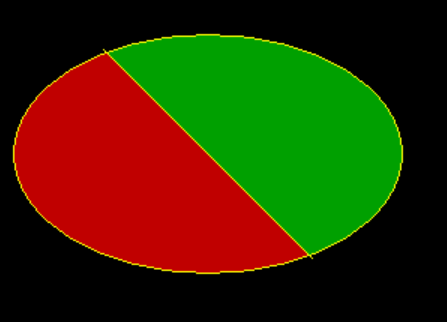GeeksforGeeks App
Open AppBrowser
Continue

# Draw an ellipse divided by straight line into two colored part in C++ Graphics

Prerequisite: How to include graphics.h?, graphics.h
The task is to draw an ellipse that is intersected by a straight line passing through the center of the ellipse diagonally. And then color both segments with different colors.

Approach: To run the program we need to include the following header file:

```#include <graphic.h>
```

We will create the desired ellipse with the help of the following functions:

Below is the implementation of the above approach:

## C++

 `// C++ program for the above approach``#include ` `// Function to set the pattern and``// color to be filled in the given``// segment``void` `fill(``int` `x, ``int` `y,``          ``int` `color, ``int` `boundary)``{  ``    ``//It will sets the current fill``    ``//pattern and fill color``  ` `    ``setfillstyle(SOLID_FILL,color);``  ` `    ``// fill the color in segment``    ``// having point (x, y) and``    ``// border color = boundary``    ``floodfill(x, y, boundary);``}` `// Function to create the ellipse``// using graphic library``void` `drawEllipse()``{``    ``// gm is Graphics mode which is a``    ``// computer display mode that``    ``// generates image using pixels` `    ``// DETECT is a macro defined in``    ``// "graphics.h" header file``    ``int` `gd = DETECT, gm, error;` `    ``// initgraph initializes the``    ``// graphics system``    ``initgraph(&gd, &gm, ``""``);` `    ``// set the color used for drawing``    ``setcolor(YELLOW);` `    ``// Center of ellipse``    ``int` `x = 150, y = 150;` `    ``// Draw complete ellipse angle will``    ``// be from 0 to 360``    ``int` `from_angle = 0, to_angle = 360;` `    ``// Radius of ellipse``    ``int` `x_rad = 130, y_rad = 80;` `    ``// Ellipse function``    ``ellipse(x, y, from_angle,``            ``to_angle, x_rad, y_rad);` `    ``// End points of a line passing``    ``// through center of above ellipse``    ``int` `x1 = 80, y1 = 80, x2 = 220, y2 = 220;` `    ``// Line for above end points``    ``line(x1, y1, x2, y2);` `    ``// Fill different color in two``    ``// parts of ellipse, choose point``    ``// (x-1, y) and (x+1, y)``    ``// because line is passing diagonally``    ``fill(x - 1, y, RED, YELLOW);``    ``fill(x + 1, y, GREEN, YELLOW);` `    ``getch();` `    ``// closegraph function closes``    ``// graphics mode and deallocates``    ``// all memory allocated by graphics``    ``closegraph();``}` `// Driver Code``int` `main()``{``    ``// Function call``    ``drawEllipse();``    ``return` `0;``}`

Output:My Personal Notes arrow_drop_up